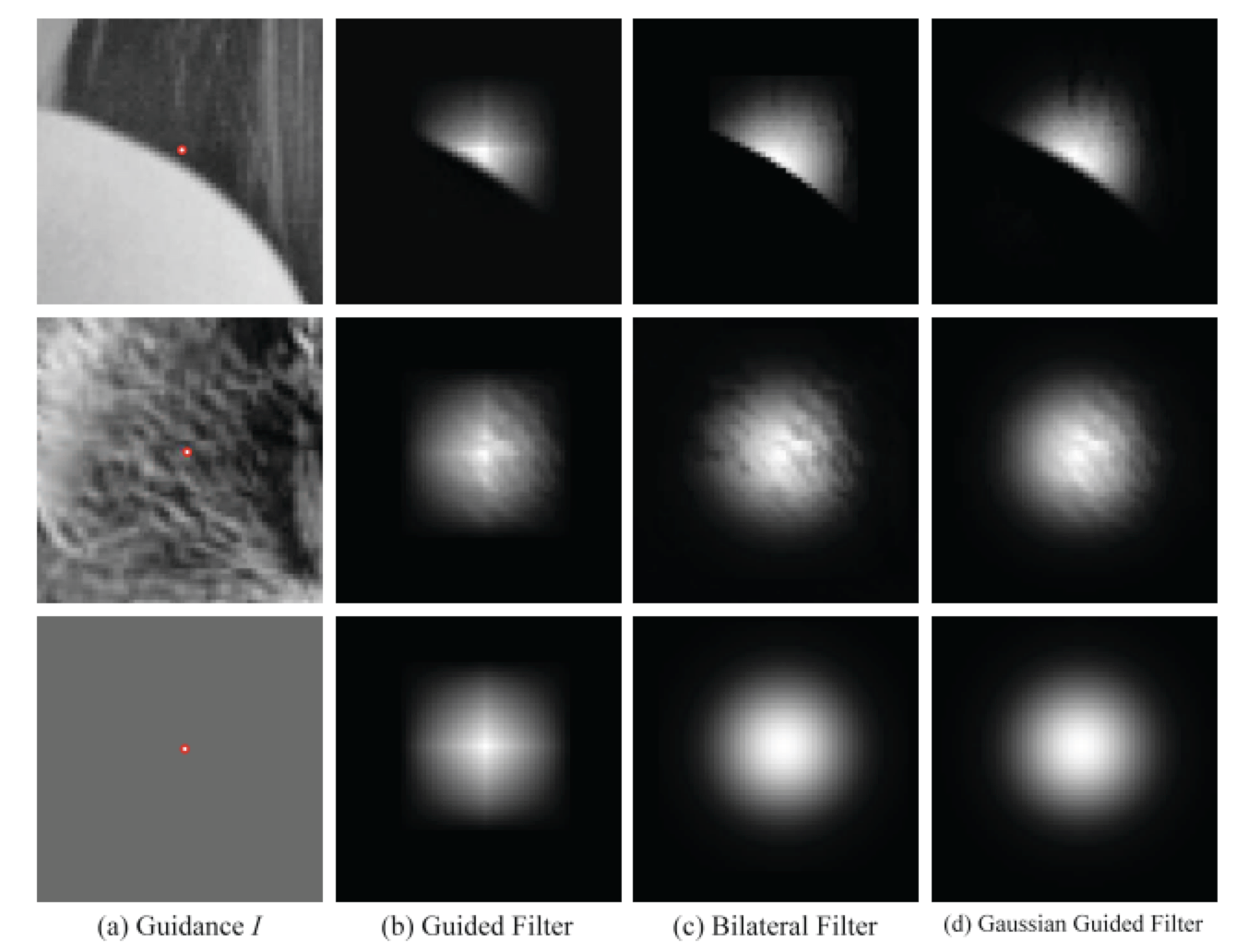# 导向滤波公式推导和扩展

#### 导向滤波的一般表达方式

$q_{i}=\sum_{j} W_{i j}(I) p_{j}$

#### 先验假设

$q_{i}=a_{k} I_{i}+b_{k}, \forall i \in \omega_{k}$

$q_{i}=p_{i}-n_{i}$

#### 能量函数和求解

$E\left(a_{k}, b_{k}\right)=\sum_{i \in \omega_{k}}\left(\left(a_{k} I_{i}+b_{k}-p_{i}\right)^{2}+\epsilon a_{k}^{2}\right)$

$a_k$$b_k$求导：
$\frac{\partial E}{\partial a_{k}}=2 \sum_{i}^{N}\left(\left(-p_{i}+a_{k} I_{i}+b_{k}\right) I_{i}+\epsilon a_{k}\right)$
$\frac{\partial E}{\partial b_{k}}=-2 \sum_{i}^{N}\left(p_{i}-a_{k} I_{i}-b_{k}\right)$

\begin{aligned} &0= \sum_{i}^{N}\left(p_{i}-a_{k} I_{i}-b_{k}\right)\\ &\Rightarrow N b_{k}=\sum_{i}^{N}\left(p_{i}-a_{k} I_{i}\right)\\ &\Rightarrow b_{k}=\frac{1}{N} \sum_{i}^{N}\left(p_{i}-a_{k} I_{i}\right)\\ &\Rightarrow b_{k}=\frac{1}{N} \sum_{i}^{N} p_{i}-a_{k} \frac{1}{N} \sum_{i}^{N} I_{i} \\ &\Rightarrow b_{k}= \bar{p_{k}}-a_{k} \mu_{k} \end{aligned}

\begin{aligned} &0= \sum_{i}^{N}\left(\left(-p_{i}+a_{k} I_{i}+b_{k}\right) I_{i}+\epsilon a_{k}\right)\\ &\Rightarrow 0=\sum-p_{i} I_{i}+\sum a_{k} I_{i}^{2}+\sum b_{k} I_{i}+\sum \varepsilon a_{k}\\ &\Rightarrow \sum p_{i} I_{i}-\sum b_{k} I_{i}=a_{k} \sum\left(I_{i}^{2}+\epsilon\right)\\ &\Rightarrow \sum p_{i} I_{i}-\sum\left(p_{k}-a_{k} \mu_{k}\right) I_{i}=a_{k} \sum\left(I_{i}^{2}+\epsilon\right)\\ &\Rightarrow a_{k}=\frac{\sum\left(p_{i} I_{i}-p_{k} I_{i}\right)}{\sum\left(I_{i}^{2}-\mu_{k} I_{i}+\epsilon\right)}\\ &\Rightarrow a_{k}=\frac{\sum\left(p_{i} I_{i}\right)-\frac{1}{N} \sum p_{i} \sum I_{i}}{\sum I_{i}^{2}-\frac{1}{N} \sum I_{i} \sum I_{i}+\epsilon}=\frac{\frac{1}{N} \sum\left(p_{i} I_{i}\right)-\frac{1}{N} \sum p_{i} \frac{1}{N} \sum I_{i}}{\frac{1}{N} \sum I_{i}^{2}-\frac{1}{N} \sum I_{i} \frac{1}{N} \sum I_{i}+\epsilon} \end{aligned}

\begin{aligned} &\operatorname{var}(X)=\frac{\sum_{i=1}^{n}\left(X_{i}-\bar{X}\right)^2}{n-1}\\ &\operatorname{Cov}(X, Y)=E[(X-E[X])(Y-E[Y])]\\ &\operatorname{Cov}(X, Y)=E[X Y]-E[X] E[Y] \end{aligned}

$a_{k}=\frac{\operatorname{Cov}(p, I)}{\operatorname{Var}(I)+N \epsilon}=\frac{\operatorname{Cov}(p, I)}{\sigma_{k}^{2}+\epsilon}$

\begin{aligned} a_{k} &=\frac{\sigma_{k}^{2}}{\sigma_{k}^{2}+\varepsilon} \\ b_{k} &=\left(1-a_{k}\right) \mu_{k} \end{aligned}

• 当遇到边缘区域，方差较大，此时$\sigma_{k}^2>>\epsilon$，有$q=p$
• 反之，当方差较小，$\sigma_{k}^2<<\epsilon$，输出均值$q=\mu_k$

#### 扩展

##### 简化计算

$q_{i}=\bar{a}_{i} I_{i}+\bar{b}_{i}$

##### 滤波核计算

$q_{i}=\frac{1}{|\omega|} \sum_{k \in \omega_{i}}\left(a_{k}\left(I_{i}-\mu_{k}\right)+\bar{p}_{k}\right)$
$\frac{\partial q_{i}}{\partial p_{j}}=\frac{1}{|\omega|} \sum_{k \in \omega_{i}}\left(\frac{\partial a_{k}}{\partial p_{j}}\left(I_{i}-\mu_{k}\right)+\frac{\partial \bar{p}_{k}}{\partial p_{j}}\right)$

$\frac{\partial \bar{p}_{k}}{\partial p_{j}}=\frac{1}{|\omega|} \delta_{j \in \omega_{k}}=\frac{1}{|\omega|} \delta_{k \in \omega_{j}}$

\begin{aligned} \frac{\partial a_{k}}{\partial p_{j}} &=\frac{1}{\sigma_{k}^{2}+\epsilon}\left(\frac{1}{|\omega|} \sum_{i \in \omega_{k}} \frac{\partial p_{i}}{\partial p_{j}} I_{i}-\frac{\partial \bar{p}_{k}}{\partial p_{j}} \mu_{k}\right) \\ &=\frac{1}{\sigma_{k}^{2}+\epsilon}\left(\frac{1}{|\omega|} I_{j}-\frac{1}{|\omega|} \mu_{k}\right) \delta_{k \in \omega_{j}} \end{aligned}

$\frac{\partial q_{i}}{\partial p_{j}}=\frac{1}{|\omega|^{2}} \sum_{k \in \omega_{i}, k \in \omega_{j}}\left(1+\frac{\left(I_{i}-\mu_{k}\right)\left(I_{j}-\mu_{k}\right)}{\sigma_{k}^{2}+\epsilon}\right)$

##### 高斯导向滤波

$E\left(a_{k}, b_{k}\right)=\sum_{i \in \omega_{k}} w_{i k}\left(\left(a_{k} I_{i}+b_{k}-p_{i}\right)^{2}+\epsilon a_{k}^{2}\right)$